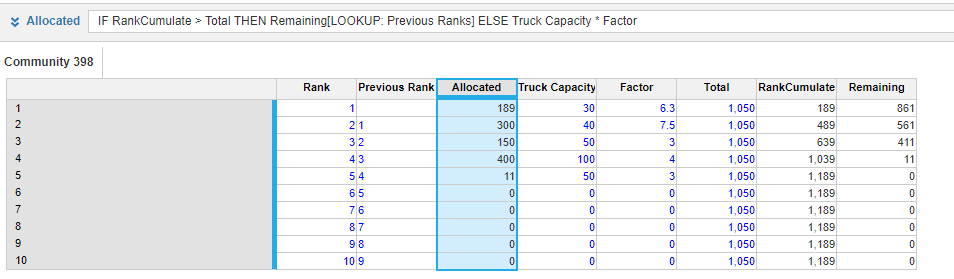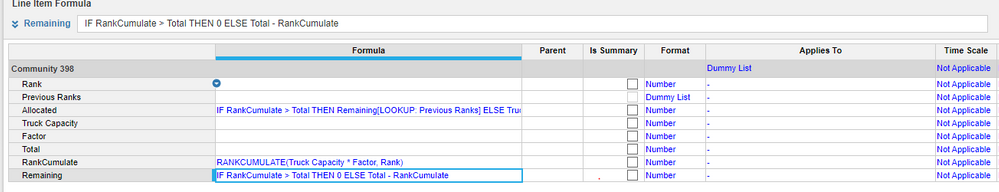# Circular Reference Issue in rank traversalI have a problem statement where I am trying to do some allocation basis rank traversal

there is fixed quantity Q= 900 which i have to distribute amongst vendors .

e.g if i allocate 100 to V1 basis some logic, then I am calculating remaining quantity for allocation to other vendors V2 where I calculating remaining quantity = Q- cumulate of allocated till now. Here I am facing issue of circular reference. I need to stop traversing whenever remaining for allocation is zero or cumulate of allocated till now equals the fixed quantity Q which we had to distribute.

Many thanks for any suggestions that I may get

Tagged:

•Explore RANKCUMULATE function. Or else if you can share some screenshots probably we can help better. Note: this function is heavy on anaplan engine and there is a upper limit on the number of cells.

•Try this let me know if it helps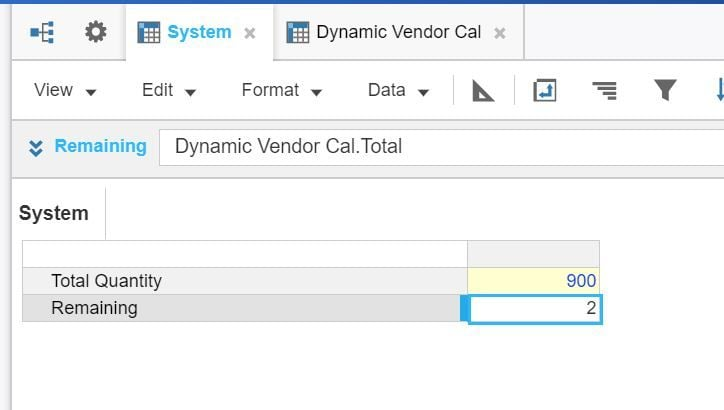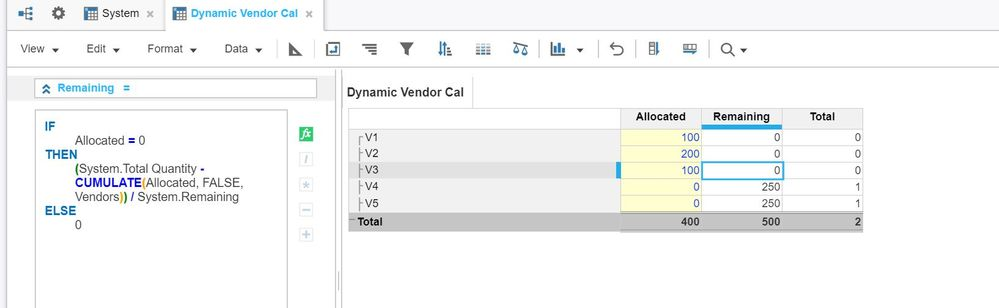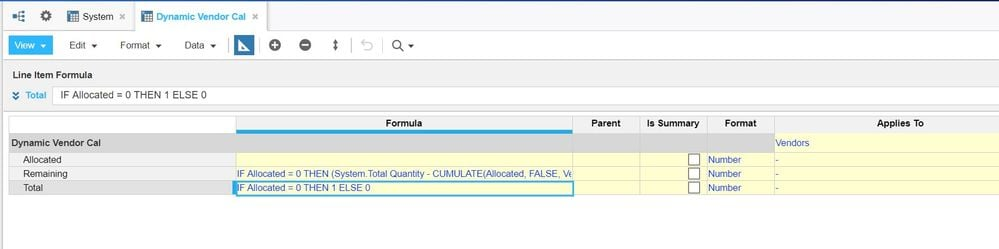Thanks,

•Thank you for the prompt response

requirement is as I traverse the vendors from V1 through V5, I will have remaining quantity calculated as per the allocation and total quantity

Hence considering your example, V1 allocated 100 , remaining should be 900-100

Similarly for V2 if allocated is 200, then remaining would be 900-100-200 and so on

Please suggest if i could give clarity

•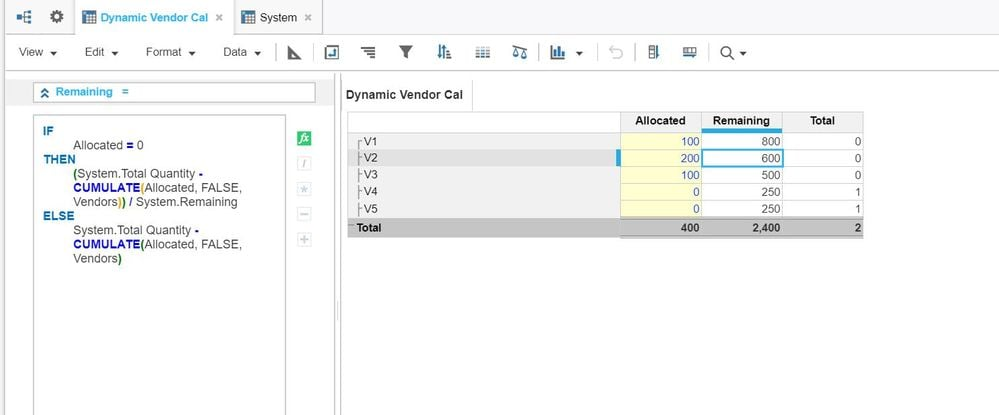Thanks,

•Hi Srishti,

Have you tried using RANKCUMULATE?

Regards,
Anirudh
•Hi Anirudh

yes I have tried rankcumulate , however when I am calculating allocated from remaining quantity and remaining from total and allocated quantity is where I am facing circular reference issue

•When I am next trying to calculate allocated from remaining quantity, is when i am facing circular reference issue.

Here I am trying to allocate remaining quantity to next vendor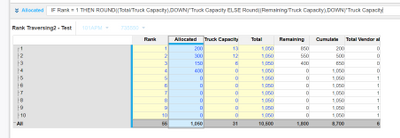•What is it that you are trying to do in your Allocated formula? If I trust my mathematical skills you are having Truck capacity in numerator as well as in denominator which will cancel each other and you ultimately have the same  "Total" or "Remaining" amount

I think you are trying to allocate the Total numbers based on the truck capacity and then burn it down until it reaches the maximum limit or is there any other calculations that goes into Allocated Line item?

See if below helps: